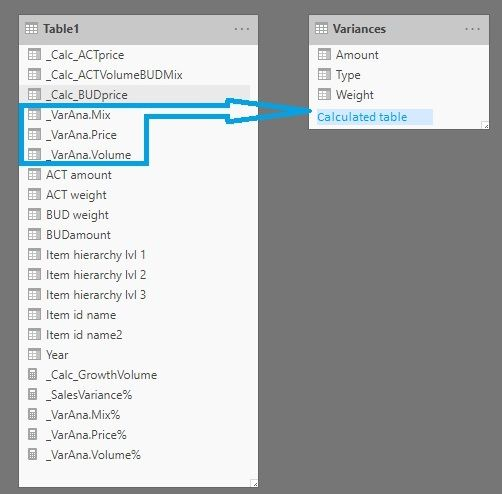cancel
Showing results for
Did you mean:Anonymous
Not applicable

## How to make a calculated table dependent on the source's slicer

Hi!

As I've tried to illustrate in the picture below I have created a calculated table (Variances) out of some fields in the main table (Table1).

The formula behind the calculated table is as follows:

``````Variances =

UNION(
Row("Type";"BUD";
"Amount";sum(Table1[BUDamount]);
"Weight";sum(Table1[BUD weight])
);
ROW("Type";"Volume";
"Amount";sum(Table1[_VarAna.Volume]);
"Weight";0
);
ROW("Type";"Mix";
"Amount";SUM(Table1[_VarAna.Mix]);
"Weight";0
);
Row("Type";"Price";
"Amount";SUM(Table1[_VarAna.Price]);
"Weight";0
);
Row("Type";"ACT";
"Amount";sum(Table1[ACT amount]);
"Weight";sum(Table1[ACT weight])
)
)``````

This works perfectly as long as I don't apply filters (slicer) to my report. The problem is that the calculated table disregards my slicer (Table[Year]). I assume I have to apply CALCULATETABLE somehow, and I've tried that (also CALCULATE) in different ways without luck.

Any suggestions as to how I can achieve a calculated table that responds to the slicer in Table1?2 REPLIES 2Community Champion

hi @Anonymous

if i understand you correct it should be enough to inclue your sum-statements into CALCULATE like

``````Variances =

UNION(
Row("Type";"BUD";
"Amount";CALCULATE(sum(Table1[BUDamount]));
"Weight";CALCULATE(sum(Table1[BUD weight]))
);
ROW("Type";"Volume";
"Amount";CALCULATE(sum(Table1[_VarAna.Volume]));
"Weight";0
);
ROW("Type";"Mix";
"Amount";CALCULATE(SUM(Table1[_VarAna.Mix]));
"Weight";0
);
Row("Type";"Price";
"Amount";CALCULATE(SUM(Table1[_VarAna.Price]));
"Weight";0
);
Row("Type";"ACT";
"Amount";CALCULATE(sum(Table1[ACT amount]));
"Weight";CALCULATE(sum(Table1[ACT weight]))
)
)``````

after that your slicer from the sae table should affect your visual

do not hesitate to give a kudo to useful posts and mark solutions as solution

do not hesitate to give a kudo to useful posts and mark solutions as solutionAnonymous
Not applicable

Thanks for your response @az38 !

I tried your modification, and unfortunately it works in an opposit direction of what I need. CALCULATE without any filter clears even the row context. I need row context to be preserved, and also have the table recalculated based on the slicer selection. I do believe CALCULATE or CALCULATETABLE is a part of the solution, but with some sort of filter included. Maybe ALLSELECTED / ALLEXCEPT, but I'm not able to figure out which and exactly how to design/write it.

I hope this clearifies, and if not please don't hesitate to ask!

Thomas